# The surprisingly good performance of dumb classification algorithms

## 140 lines of code (Python)

#### When evaluating binary classification algorithms it is a good idea to have a baseline for the performance measures. In this blog post I calculate the classification performance of really dumb classifiers. These models do not use any feature information. If your own classification model performs just like them, there is a problem.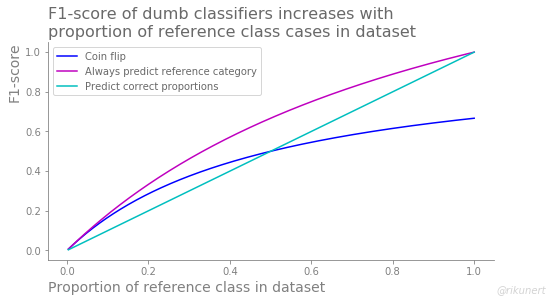## Dumb classifiers

When evaluating whether your classification model is any good, you will probably use one of these performance measures:

• precision: the proportion of true positives (actually positive cases and predicted to be positive cases) among predicted positive cases
• recall: the proportion of true positives (actually positive cases and predicted to be positive cases) among all actually positive cases
• F1-score: the harmonic mean of precision and recall
• accuracy: the proportion of correctly predicted cases among all cases

Note that precision, recall and F1-score operate with a reference category. This is usually the target category one is interested in, for example:

• The person is pregnant (as opposed to not pregnant).
• The person does convert (as opposed to not becoming a customer).
• The e-mail is spam (as opposed to legitimate).

When using these performance measures it is worth evaluating them against a baseline. What would be a good baseline? I believe dumb classifiers which bear no relation to artificial intelligence are a good start. They ignore all feature information and instead rely purely on information about the classification target.

In this blog post I focus on three dumb binary classifiers:

• Coin flip: This model flips a coin every time it sees a case. Half the cases are predicted to belong to the reference category, half do not.
• Always predict reference category: This model always, no matter what, predicts cases to be in the reference category. It’s like a coin flip where every time face is up.
• Predict correct proportions: This model knows about the proportion of the reference category in the dataset and changes its predictions accordingly. The proportion of reference category cases is the same in the actual target vector as in the predicted target vector. In all other respects, the classification is random.

## The coin flip performance

The coin flip classifier predicts half the cases as belonging to the reference category. Therefore its recall and its accuracy are always 50%. The precision score behaves completely differently for this dumb classifier. It always has the same values as the reference category proportion. As a result the F1-score correlates with the proportion of reference category cases as well, albeit less strongly.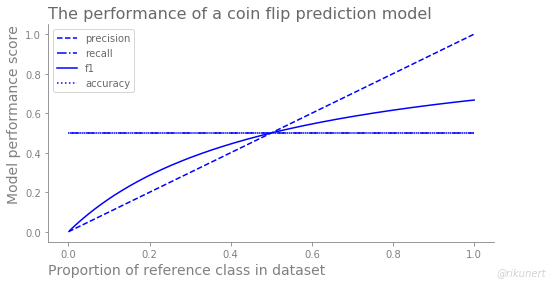## The performance of always predicting the reference category

This classifier exclusively predicts positive cases, like a pregnancy test always indicating a pregnancy. As a result, no positive case gets missed and the recall is always 100%. However, accuracy and precision hold the same value as the proportion of reference category cases in the dataset. The F1-score, being a combination of precision and recall, also correlates with the reference category proportion, albeit less strongly.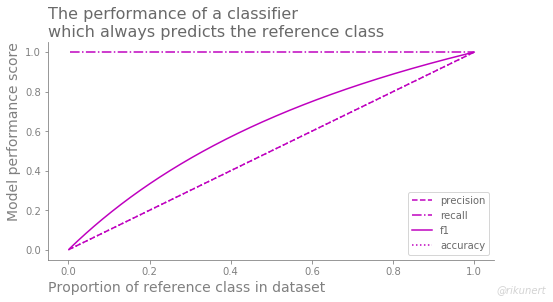## The performance of randomly predicting the right proportion of positive cases

This classifier predicts the right proportion of reference category cases but it does so randomly. As if the pregnancy test knew how many women were pregnant but not which ones. This classification strategy leads to an accuracy which is always above 50%. Precision, recall and F1-score, on the other hand, hold the same values as the proportion of reference category cases in the dataset.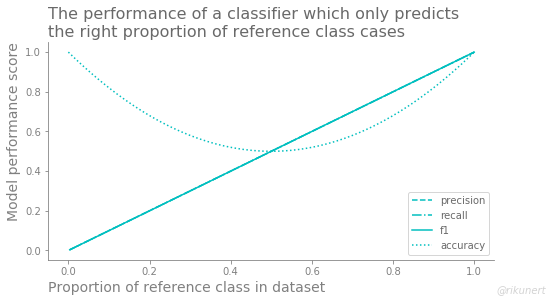## The best performance among the dumb classifiers

We can summarise these performancy measures individually to get a better overview. What is a good precision baseline? The answer is simple: the proportion of positive cases in the dataset.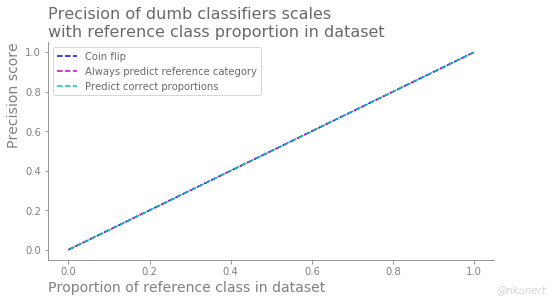What is a good recall baseline? This entirely depends on the classifier strategy.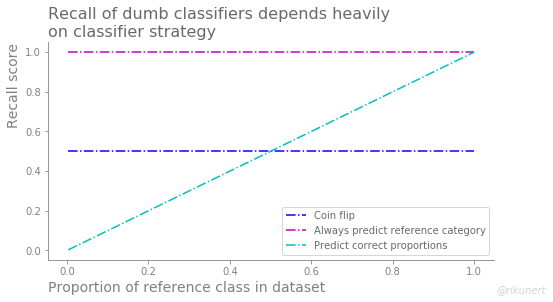What is a good F1-score baseline? This also depends on the classifier strategy but in general the F1-score increases with the proportion of positive cases in the dataset.What is a good accuracy score baseline? This also depends on the classifier strategy. Surprisingly though, many dumb classifiers do not fall below 50%.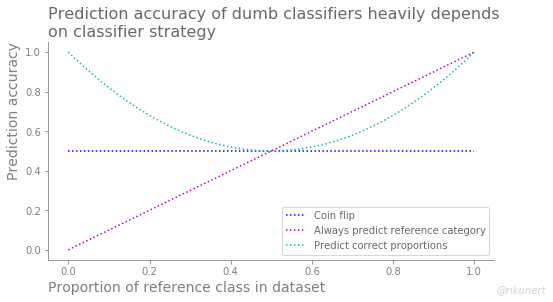The best performance for each proportion of the reference class is summarised in this figure: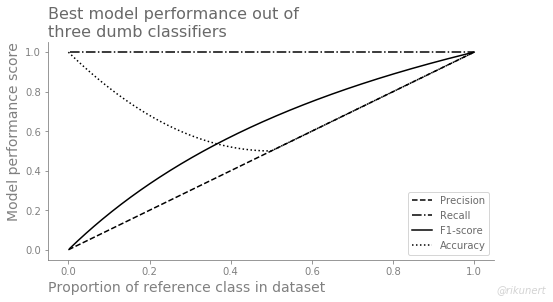Note how recall and accuracy are difficult to interpret as the baseline formed by dumb classifiers is already very high.

## Conclusion

The take-home message of this look at dumb classifiers is that no one performance measure is enough to properly evaluate the performance of a model. Random predictions can lead to surprisingly high performance measures, especially for recall and accuracy. Better to look at a number of them to get a full picture. This way you can trust your binary classifier and its predictions.

The complete code to recreate the analyses and plots of this blog post can be found on github here.